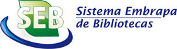Autor
 Palavra-chave
 Tipo do documento
 Ano
 País
 Idioma
 Ordenar por: Relevância Autor Título AnoPrimeira ... 1 ... ÚltimaNwakuba, Nnaemeka Reginald. Development of a mathematical model for predicting the drying rate of a thin layer cocoa bean in a convective hot air dryer using dimensional analysis based on the Buckingham’s π theorem is presented. Results obtained show that drying rate decreases as the moisture level reduces due to increase in the intra-particle resistance to internal moisture migration of the cocoa beans. High coefficients of determination of 94.7%, 94.7% and 99.5% at temperatures of 60, 70 and 80oC respectively between the predicted and experimental values showed that the method is good. The model was validated with data obtained from an existing thin layer convective dryer and there was no significant difference between the experimental drying rate and the predicted values at 5%... Tipo: Info:eu-repo/semantics/article Palavras-chave: Agric. Engineering; Crop Processing engineering cocoa bean; Thin layer drying; Dimensional analysis; Drying rate; Prediction equation.. Ano: 2017 URL: http://www.cigrjournal.org/index.php/Ejounral/article/view/4107Günther,Bruno; Morgado,Enrique. A wide spectrum of cyclic functions in terrestrial mammals of different size, from the 3-gram shrew to the 3-ton elephant, yields an allometric exponent around 0.25, which is correlated _ as a kind of common denominator _ with the specific metabolic rate. Furthermore, the applicability of these empirical findings could be extrapolated to chronological events in the sub-cellular realm. On the other hand, the succession of growth periods (T98%) until sexual maturity is reached also follows the 1/4 power rule. By means of Verhulst's logistic equation, it has been possible to simulate three different biological conditions, which means that by modifying the numerical value of only one parameter, revertible physiological and pathological states can be obtained,... Tipo: Journal article Palavras-chave: Growth; Dimensional analysis; Specific metabolic rate; Logistic equation; Homeostasis. Ano: 2005 URL: http://www.scielo.cl/scielo.php?script=sci_arttext&pid=S0716-97602005000200010GÜNTHER,BRUNO; MORGADO,ENRIQUE. ABSTRACT The aim of the present study was to compare two different biological similarity criteria, one was based on body mass (M) as a theoretical reference system in accordance with the MLT-system of physics, while the other utilized the body weight (W) for the same purpose. The mass-dependent allometry should be applied during space flights as well as during fetal and newborn conditions of life, whereas the weight-dependence should prevail in earth-bound physiology. The above mentioned distinctions are relevant not only for the specific metabolic rates but also for numerous biological time functions, as for instance for the heart and respiratory rates of all mammals, whose allometric exponent is b = - 0.09 during fetal life, and b = - 0.25 in all adult... Tipo: Journal article Palavras-chave: Dimensional analysis; Biological similarity; Allometry; Biological time; Fetal life; Space physiology. Ano: 2003 URL: http://www.scielo.cl/scielo.php?script=sci_arttext&pid=S0716-078X2003000100006GÜNTHER,BRUNO; MORGADO,ENRIQUE. The applicability of dimensional analysis (DA) is discussed in relation to the metabolic scaling laws. The evolution of different theories of biological similarity has shown that the calculated reduced exponents (b) of Huxley's allometric equation are closely correlated with the numerical values obtained from the statistical analysis of empirical data. Body mass and body weight are not equivalent as biological reference systems, since in accordance to Newton's second law, the former has a dimension of a mass, while the latter should be dimensionally considered as a force (W = MLT-2). This distinction affects the coefficients of the mass exponent (a). This difference is of paramount importance in microgravity conditions (spaceflight) and of buoyancy during... Tipo: Journal article Palavras-chave: Dimensional analysis; Allometric scaling; Theories of biological similarity; Body mass; Body weight. Ano: 2003 URL: http://www.scielo.cl/scielo.php?script=sci_arttext&pid=S0716-97602003000300011GÜNTHER,BRUNO; MORGADO,ENRIQUE; JIMÉNEZ,RAÚL F. In the present paper we have examined the applicability of dimensionless and invariant numbers (DN & IN) to the analysis of the cardiovascular system of mammals, whose functions were measured at standard metabolic conditions. The calculated IN did not change when we compared these figures with those obtained in dogs while they were submitted to graded exercise on a treadmill. In both instances, rest and exercise, the constancy of the IN prevailed, in accordance with Cannon's principle of "homeostasis" (1929). On the contrary, when dogs were examined during a standardized hypovolemic shock, we observed a breakdown of the IN, and the resulting DN evolved as a reliable index of the condition of "heterostasis" as defined by H. Selye. The robustness of... Tipo: Journal article Palavras-chave: Dimensional analysis; Biological similarities; Allometric equations; Rest and exercise; Hemorrhagic shock; Integral feedback. Ano: 2003 URL: http://www.scielo.cl/scielo.php?script=sci_arttext&pid=S0716-97602003000200011Bombardelli,Wagner W. Á.; Camargo,Antonio P. de; Frizzone,José A.; Lavanholi,Rogério; Rocha,Hermes S. da. ABSTRACT Information about local head loss caused by connections employed in micro-irrigation systems is hard to be found in literature. The objective of this research was to experimentally determine the local head losses in connections commonly used in micro-irrigation and propose mathematical models using the theorem of Buckingham. The methodology of tests was based on international standards. The tests were carried out under controlled inlet pressure, at 150 kPa, and five to ten units of each connection model were tested. The curves relating flow and head losses were drawn based on 15 flow conditions, obtained under increase and decrease of flow rate. For each condition, 30 points were collected resulting in a sample size of 900 points in each test. For... Tipo: Info:eu-repo/semantics/article Palavras-chave: Hydraulics; Dimensional analysis; Buckingham Pi theorem. Ano: 2019 URL: http://www.scielo.br/scielo.php?script=sci_arttext&pid=S1415-43662019000700492NESPOLO,ROBERTO F. The necessity of the mathematical description of living processes and the recent advances in theoretical syntheses that fit surprisingly well with real data have led many scientists to experiment with new "generalizations," beginning with allometric equations. I present here, briefly, why caution is needed when allometric equations are multiplied or divided to make new ones. This practice is flawed by old and recognized problems, such as the fallacy of averages combined with misunderstandings that include error propagation, misuse of statistics and confounding scale. Tipo: Journal article Palavras-chave: Fallacy of averages; Dimensional analysis; Invariants; Allometric equations; Error term. Ano: 2005 URL: http://www.scielo.cl/scielo.php?script=sci_arttext&pid=S0716-97602005000100004